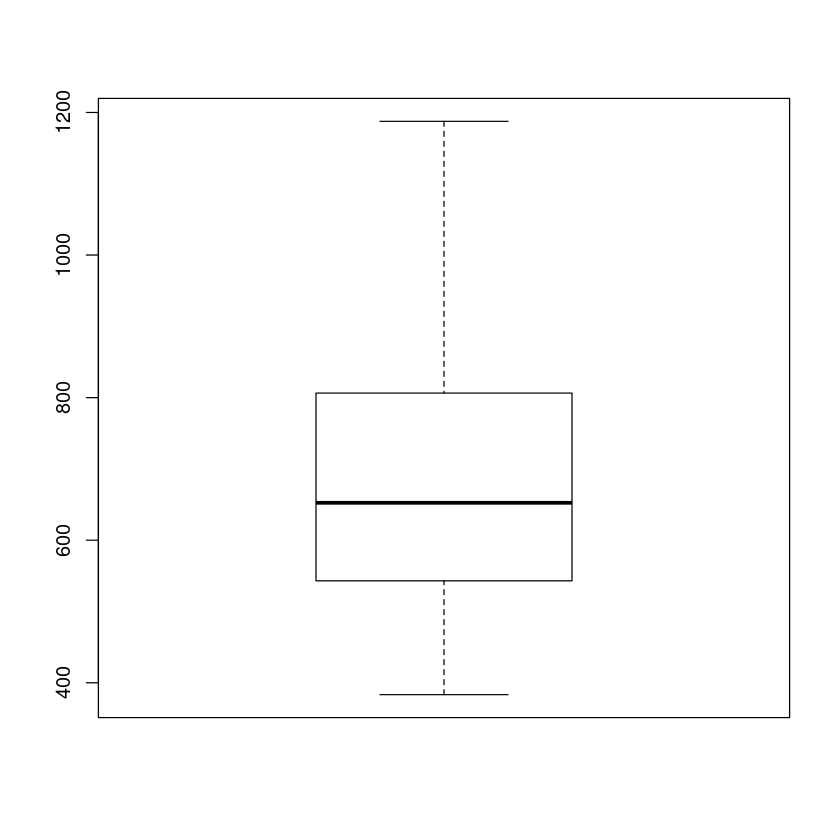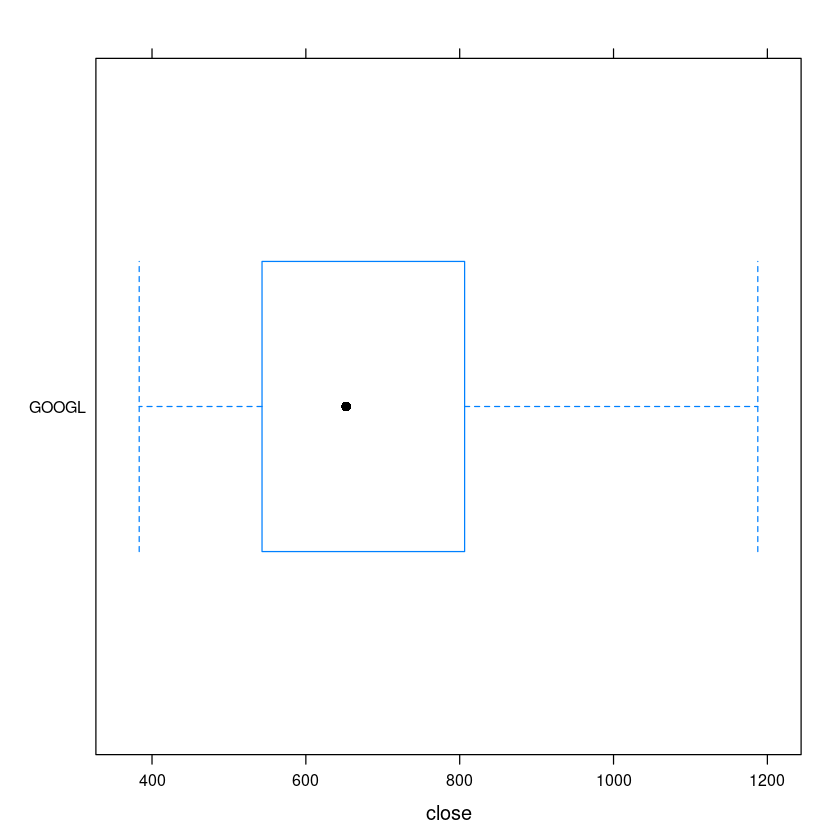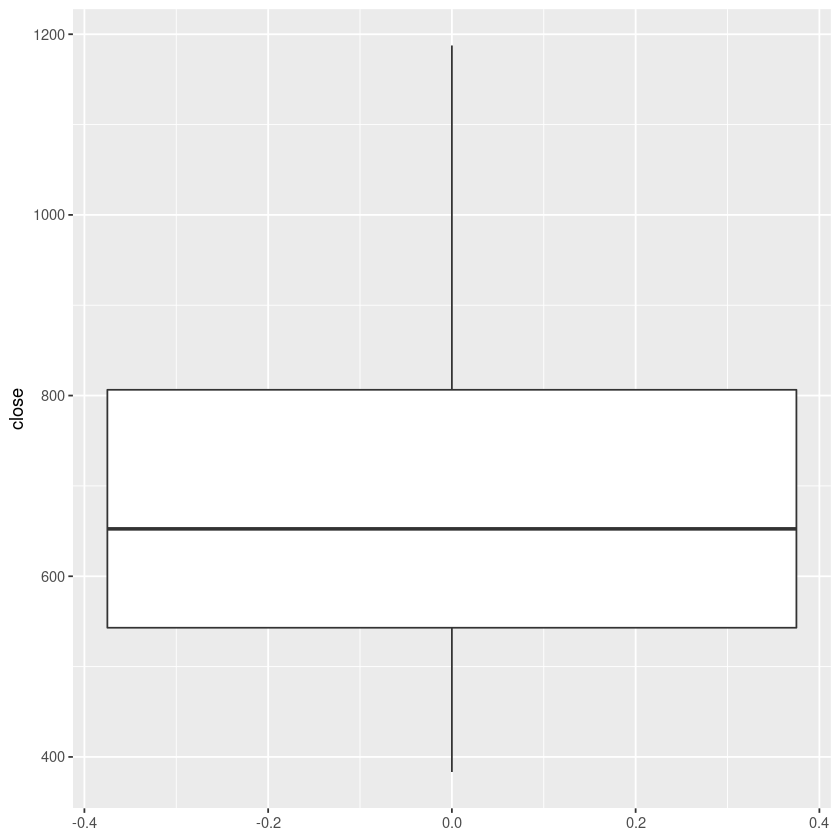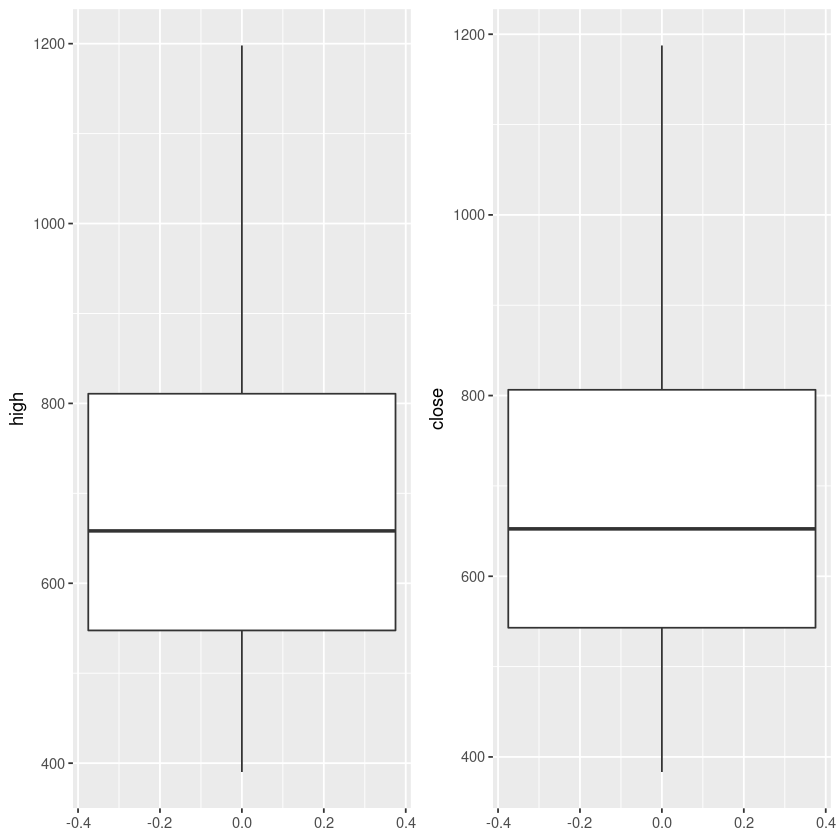# Top Non Javascript Boxplot Libraries In R With Examples

Last Updated 2020-05-17

In this tutorial, i will talk about some of the best libraries for drawing boxplots in R.

1. boxplot() R native
2. bwplot() Lattice
3. ggplot() Ggplot2

For this tutorial, I will use stocks data which i downloaded from Kaggle.

Let us read our csv file for stock Google and convert the data in to dataframe.

In :
stock.data <- read.csv('data/individual_stocks_5yr/individual_stocks_5yr/GOOGL_data.csv')
stock.data.df <- data.frame(stock.data)

In :
head(stock.data.df,2)

A data.frame: 2 × 7
dateopenhighlowclosevolumeName
<fct><dbl><dbl><dbl><dbl><int><fct>
12013-02-08390.4551393.7283390.1698393.07776031199GOOGL
22013-02-11389.5892391.8915387.2619391.60124330781GOOGL

## R Boxplot

boxplot() is native library in R and it is very easy to use. Checkout boxplots in R for more details.

In :
boxplot(stock.data.df\$close)## Boxplot using Lattice

If you don't have it installed, install it using install.package('lattice') in R repl or R studio.

In :
library(lattice)


We can draw the plot using bwplot() function.

In :
bwplot(Name~close,data=stock.data.df)We can rotate the plot, if we reverse the order in bwplot() function as shown below.

In :
bwplot(close~Name,data=stock.data.df,ylab='Google Close Price')## Boxplot in Ggplot2

Install ggplot2 in R repl or Rstudio with install.packages('ggplot2')

In :
library(ggplot2)

In :
ggplot(stock.data, aes(y=close)) +
geom_boxplot()In :
ggplot(stock.data, aes(y=close)) +
geom_boxplot(notch = TRUE) + coord_flip()We can draw multiple box plots side by side with package gridExtra. Install it using install.packages('gridExtra')

In :
library(gridExtra)
gghigh <- ggplot(stock.data.df, aes(y=high)) +  geom_boxplot()
ggclose <- ggplot(stock.data.df, aes(y=close)) +  geom_boxplot()
grid.arrange(gghigh,ggclose,ncol=2)## Wrap Up!

Let me know if you know of any good R non javascript library packages by emailing me at johnludhi at outlook.com. I will cover it here.